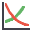The Rocscience International Conference 2021 Proceedings are now available. Read Now

# Sensitivity Analysis

The effect of uncertainty or variability in the values of input parameters can be explored using a Sensitivity Analysis. In sensitivity analysis, model parameters selected by the user are varied across a range of values and the effect on safety factor observed. This exercise helps identify the parameters that have the most effect on the stability of a crown pillar, and can be used to compare the effectiveness of various remedial measures.

The results of a sensitivity analysis are displayed on a sensitivity plot, which plots the safety factor against percent change in the input variable(s).

On a sensitivity plot, the gradient of a curve for a parameter indicates the effect that parameter has on the factor of safety. Steeper (rising or falling) curves indicate greater influence on the factor of safety. A relatively "flat" curve indicates that a variable has little effect on the factor of safety. A completely flat curve indicates that the variable has NO effect on the safety factor (within the specified range of the variable).

To carry out a Sensitivity Analysis with CPillar:

1. Select the Sensitivityoption from the toolbar or Analysis menu.
2. You will see the Sensitivity Analysis Input dialog.
3. Select the Add button for the number of variables you would like to plot (e.g. if you would like to plot 3 variables select Add three times).
4. Default variables are automatically loaded. To choose the variables you would like to plot, click on a variable name. A drop-list of all possible variables will appear, from which you can select a desired variable. Do this for each variable.

NOTE: If you do not see a variable you would like to plot, then this means it has not yet been defined in the model.

1. Using the From and To input edit boxes, enter a range within which you would like each parameter to vary.
2. To display the current input values (i.e.. the mean values from the main input data) select the Show current project input values check box. This is useful as a guide for defining your range of values for each parameter.
3. When you are done entering parameters, click the Plot button.
4. On a single graph, CPillar will generate the specified sensitivity plot(s).

NOTES:

• A Sensitivity Plot in CPillar is generated by subdividing each variable range (defined by the From and To values in the Sensitivity Input dialog), into 50 intervals, and calculating the safety factor at each value of the variable between the From and To values. If you right-click on the graph, and select the Vertex Markers option, you will see the actual points used to generate the curve(s).
• Remember that each curve on a Sensitivity Plot is obtained by varying only ONE PARAMETER, while keeping all other variables constant (at their mean value).
• The Percent of Range (on the horizontal axis of a Sensitivity Plot) is the relative difference between the Minimum value of a variable (0 percent) and the Maximum value of a variable (100 percent), according to the From and To ranges you have entered in the Sensitivity dialog.
• If you are only plotting a single variable on a Sensitivity Plot, then the horizontal axis will be in terms of the actual value of the variable, rather than Percent of Range.

## Right-ClickOptions

Several options are available if you right-click on a Sensitivity plot. For example:

• You can modify the data in an existing Sensitivity Plot, by right-clicking the mouse in the plot, and selecting Change Plot Data. This will display the Sensitivity Input dialog with the current plot variables.
• Select the Chart Properties option to customize the appearance of the plot.
• The Sampler option allows you to graphically determine the coordinates of any points on the Sensitivity Plot curves. For more information, see the discussion of the Sampler for the Cumulative Plot, as the Sensitivity Plot Sampler operates in a similar manner.